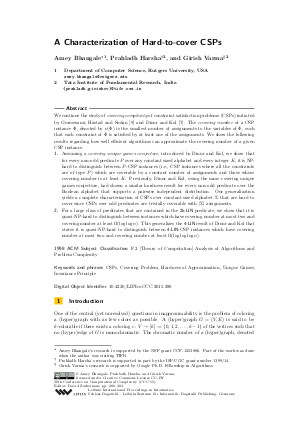Document# A Characterization of Hard-to-cover CSPs

### Authors Amey Bhangale, Prahladh Harsha, Girish Varma## File

LIPIcs.CCC.2015.280.pdf
• Filesize: 0.65 MB
• 24 pages

## Cite As

Amey Bhangale, Prahladh Harsha, and Girish Varma. A Characterization of Hard-to-cover CSPs. In 30th Conference on Computational Complexity (CCC 2015). Leibniz International Proceedings in Informatics (LIPIcs), Volume 33, pp. 280-303, Schloss Dagstuhl - Leibniz-Zentrum für Informatik (2015)
https://doi.org/10.4230/LIPIcs.CCC.2015.280

## Abstract

We continue the study of covering complexity of constraint satisfaction problems (CSPs) initiated by Guruswami, Håstad and Sudan [SIAM J. Computing, 31(6):1663--1686, 2002] and Dinur and Kol [in Proc. 28th IEEE Conference on Computational Complexity, 2013]. The covering number of a CSP instance Phi, denoted by nu(Phi) is the smallest number of assignments to the variables of Phi, such that each constraint of Phi is satisfied by at least one of the assignments. We show the following results regarding how well efficient algorithms can approximate the covering number of a given CSP instance. 1. Assuming a covering unique games conjecture, introduced by Dinur and Kol, we show that for every non-odd predicate P over any constant sized alphabet and every integer K, it is NP-hard to distinguish between P-CSP instances (i.e., CSP instances where all the constraints are of type P) which are coverable by a constant number of assignments and those whose covering number is at least K. Previously, Dinur and Kol, using the same covering unique games conjecture, had shown a similar hardness result for every non-odd predicate over the Boolean alphabet that supports a pairwise independent distribution. Our generalization yields a complete characterization of CSPs over constant sized alphabet Sigma that are hard to cover since CSPs over odd predicates are trivially coverable with |Sigma| assignments. 2. For a large class of predicates that are contained in the 2k-LIN predicate, we show that it is quasi-NP-hard to distinguish between instances which have covering number at most two and covering number at least Omega(log(log(n))). This generalizes the 4-LIN result of Dinur and Kol that states it is quasi-NP-hard to distinguish between 4-LIN-CSP instances which have covering number at most two and covering number at least Omega(log(log(log(n)))).
##### Keywords
• CSPs
• Covering Problem
• Hardness of Approximation
• Unique Games
• Invariance Principle

## Metrics

• Access Statistics
• Total Accesses (updated on a weekly basis)
0